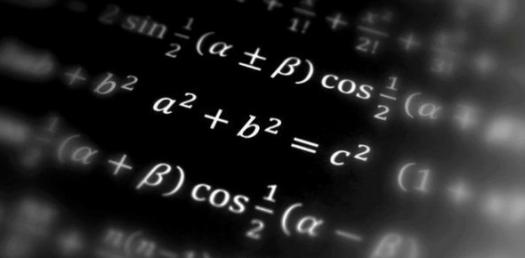# Maths Quiz (Beginner Class)

Approved & Edited by ProProfs Editorial Team
The editorial team at ProProfs Quizzes consists of a select group of subject experts, trivia writers, and quiz masters who have authored over 10,000 quizzes taken by more than 100 million users. This team includes our in-house seasoned quiz moderators and subject matter experts. Our editorial experts, spread across the world, are rigorously trained using our comprehensive guidelines to ensure that you receive the highest quality quizzes.
| By Jdude36
J
Jdude36
Community Contributor
Quizzes Created: 1 | Total Attempts: 415
Questions: 5 | Attempts: 415SettingsMath is a challenging subject and if one wants a way of refreshing their minds then one math problem or two is exactly what is needed. There are different ways that a beginner can perfect his/her skills when it comes but the most common one is through practice. Do you think you can handle this quiz for beginners? Give it a try!

• 1.

### 3x9

• A.

27

• B.

18

• C.

36

A. 27
Explanation
There's a trick to the 9 times table, starting from 9, add 1 ten and take one unit. Do that to 9, it's 18. That's 2 times 9. Then for 3 times, do it again. The answer is 27.

Rate this question:

• 2.

### 9 Tick the sums that make 9.

• A.

3 + 6

• B.

10 - 1

• C.

3x2

A. 3 + 6
B. 10 - 1
Explanation

For answer B, take away 1 from 10.

Rate this question:

• 3.

### Type in the easiest possible way to get to 6.

3 + 3
3+3
Explanation
3 add 3 is the most simple because you simply add 3 onto 3.

Rate this question:

• 4.

### The highest 2 digit number is 99. True or False?

• A.

True

• B.

False

A. True
Explanation
The highest 2-digit number is 99 because a 2-digit number can have a maximum value in the tens place of 9 and a maximum value in the ones place of 9. Therefore, the highest possible combination is 99, making the statement true.

Rate this question:

• 5.

### I am thinking of a number... It is half of 12 and double of 3.

• A.

4

• B.

6

• C.

8

B. 6
Explanation
The given statement states that the number is half of 12 and double of 3. Half of 12 is 6 and double of 3 is also 6. Therefore, the number that satisfies both conditions is 6.

Rate this question:

Related TopicsBack to top